# Moving Sum?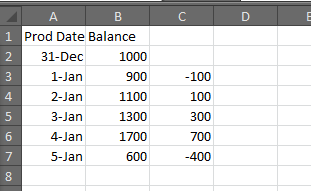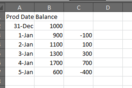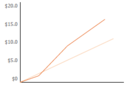Hey,

I am trying to find a calculation for column C.

Each date we have a balance.  That balance is subtracted from the previous quarter end balance.  Is this possible to do?

The finished product is the chart shown.  The dark red line is column Column C.  The x axis is column A.  For now we can ignore the light orange chart.

Thanks,

2replies Oldest first
• Oldest first
• Popular•Hi  a graz As the above result is not a straightforward calculation, we need to go through a workaround to get to the result.

First of all, we need a table which contains the Last quarter end date and balance. Then, we will join this with the existing table/Aggregated worksheet containing the values for ‘Prod Date’ and ‘Balance’ and create a formula on top of it to get the desired result.

You can follow the following steps which I have created on a sample database-

1. Create an aggregated Worksheet out of the selected columns and the formula for last quarter end date(Images 1, 2 & 3).
2. Import/Create another table which contains the quarter end dates and the respective ‘Balance’ (I have only taken data from 01/01/2016 to 03/08/2017 - Image4).
3. Join this newly created/imported table with the existing Aggregated worksheet on the Column ‘Last Quarter Date’. (Note - only inner joined values will be seen - Image5)
4. Create a formula for the Balance difference(Image 6 & 7).

If the data is large, I will rather suggest importing this column as part of ETL instead of the above steps to make the performance faster.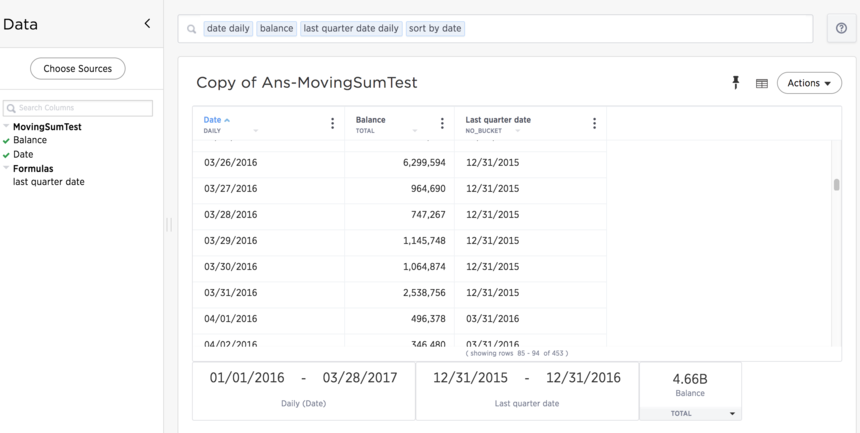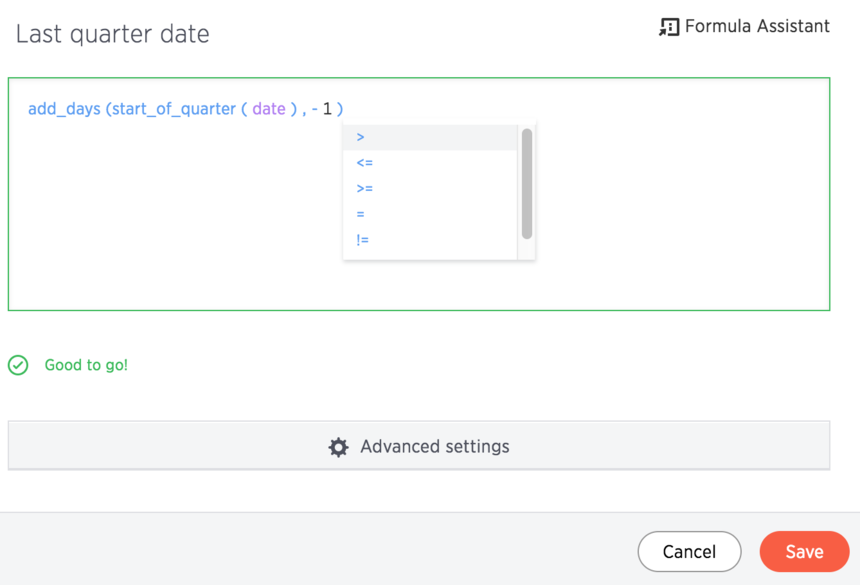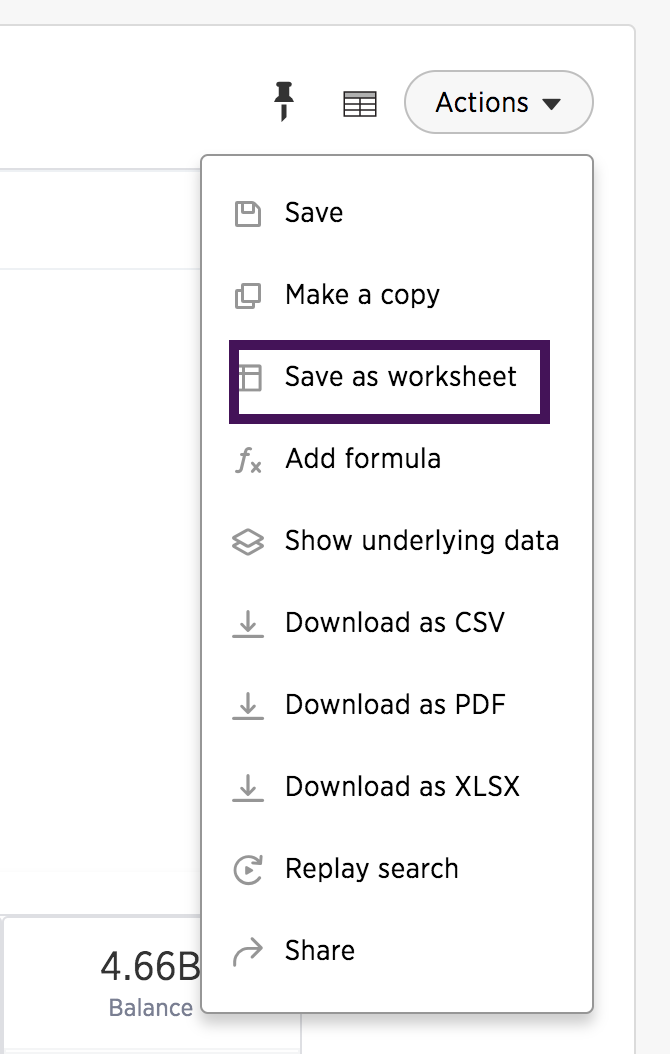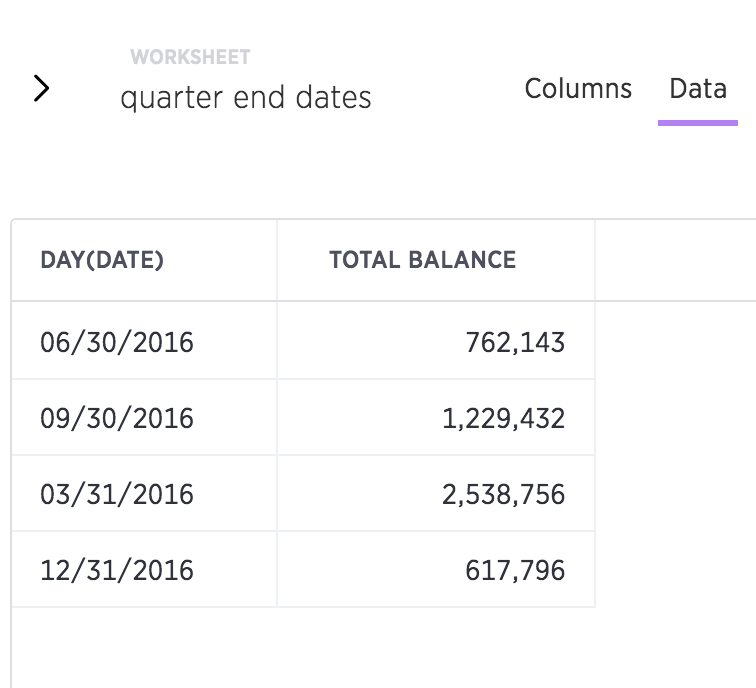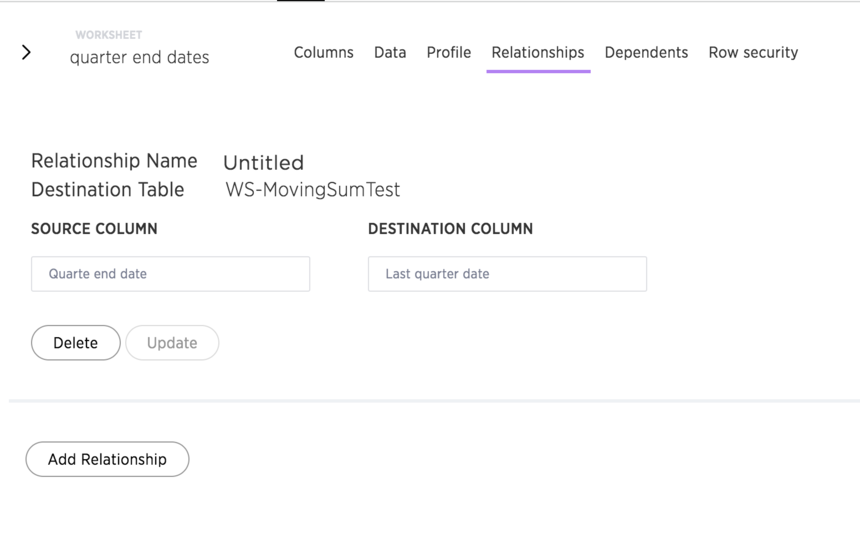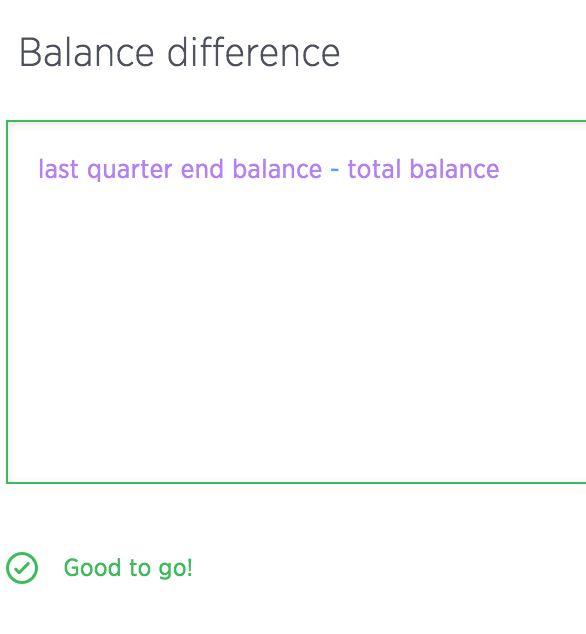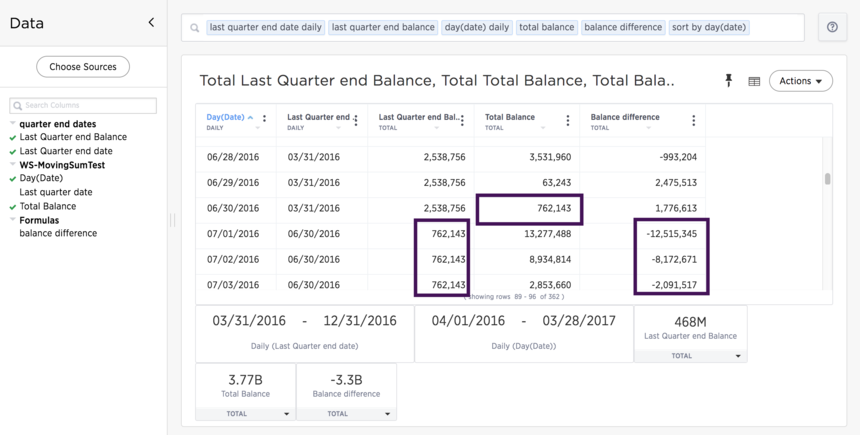Like
•Thanks Nikhita Chandra I will take a look at making these changes.

Like
Like Follow